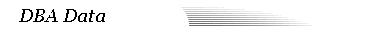[Home] [Help]

##### Line 429: SELECT distinct language FROM fnd_lookup_values

425: ,lookupCode IN VARCHAR2
426: )
427: IS
428: CURSOR csr_installed_langs IS
429: SELECT distinct language FROM fnd_lookup_values
430: WHERE lookup_type=lookupType;
431:
432: lang fnd_languages.language_code%TYPE;
433: l_string VARCHAR2(20) := 'DELETE_FND_LOOKUPS';

##### Line 464: DELETE fnd_lookup_values

460: BEGIN
461:
462: if lookupCode is null then
463:
464: DELETE fnd_lookup_values
465: WHERE lookup_type=lookupType
466: AND language = lang;
467:
468: -- Also deleting the lookup type as we have deleted all the values

##### Line 474: DELETE fnd_lookup_values

470: WHERE lookup_type=lookupType;
471:
472: else
473:
474: DELETE fnd_lookup_values
475: WHERE lookup_type=lookupType
476: AND lookup_code = lookupCode
477: AND language = lang;
478: